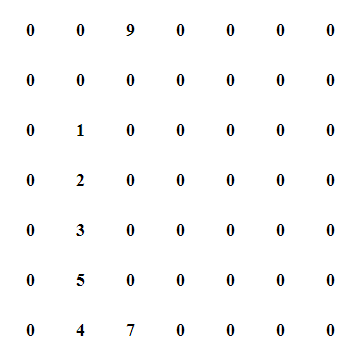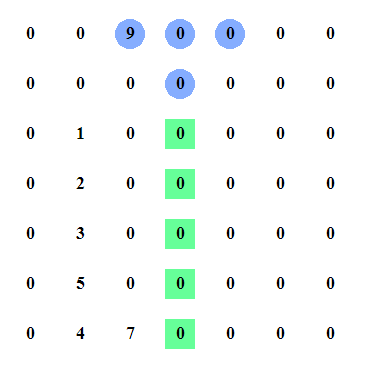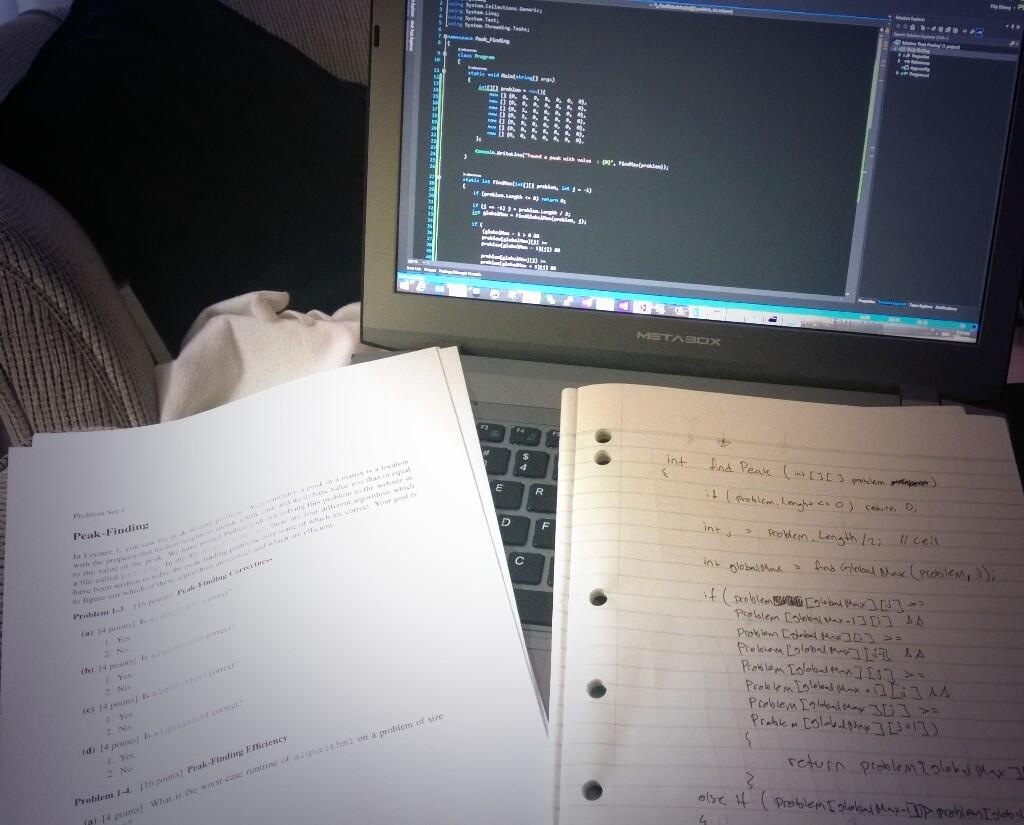# Understanding Peak-Finding

## Posted by Filip Ekberg on 10 Feb 2014

No matter how far we are in our careers as professional developers, it's great to freshen up on our fundamentals. Be it the importance of Memory Access Patterns or algorithms in general, it's really beneficial. I find it quiet interesting that it's been a pretty long time since I sat in the algorithms and data structures course on my technical institute and I tend to understand it completely different now. I heard a really great thing from a professor at MIT who said the following:

You can practice really hard for two years to become a great programmer and you can practice for 10 years to become an excellent programmer. Or you can practice for two years and take an algorithms course and become an excellent programmer

A lot of us might not think about the daily algorithms and data structures that we use, in fact, we hide behind ORMs and such that hides complexity and introduces behavior that we might not be aware of. Which is one of the reasons I personally like to read up on algorithms from time to time. This time though, I've decided to share some of the things I learn and like, hopefully you'll like it and it will help you to become an excellent programmer.

If you haven't seen this, MIT has a website called Open Courseware where they have video recordings, lecture notes, assignments and exams from their courses. There is one in particular which I recently found and it's excellent so far, it's called Introduction to Algorithms. If it's been a while since you looked into these topics, have a look at their content. Some of the examples and snippets here are from the lectures in this particular course.

Let's get to it! Back to basics!

### What is Peak-Finding?

Imagine you have a set of numbers, these numbers are stored in a one dimensional array; hence a normal array. Now you want to find one of the elements where the element peaks. Notice that we don't want to find the highest peak, we just want to find a peak. As to any problem there are multiple solutions and these solutions might differentiate from one and another. Some might be faster and some might be slower.

Let's say that we have the following set of numbers: `{1, 3, 4, 3, 5, 1, 3}`

How would you find the peak in that?

First we need to define the requirements for it to be a peak: The element needs to be larger or equal to both the elements on its sides

There's one really obvious way to solve this, can you think of it?

#### Finding the peak in one dimension (slow) O(n)

How about if we just iterate over each element and make sure that the elements surrounding it are less or equal? It's a simple solution, but is it the best and fastest? Remember that we just need to find if there is a peak somewhere in the array, it doesn't have to be the highest point.

I won't bother with showing the code for this one, it's just a simple loop with some boundary checks. The problem here is that we need to look at every element in the collection, which makes the time to run the algorithm grow linear with the growth of `n`.

#### Finding the peak in one dimension (fast) O(log n)

As the heading says, this is logarithmic, base 2 logarithmic to be exact. This means that somewhere in our algorithm we are dividing the set in two and doing so as `n` grows. So what might this mean, in terms of solving the problem? We're taking a divide and conquer approach! Just as you would with binary search. Binary search divides the array in half until it finds the correct element. Searching a phone book with 2^32 amount of records would take only 32 tries because we know it is sorted!

The same approach is applicable for the peak finding. If we take a look at the set of numbers we have again: `{1, 3, 4, 3, 5, 1, 3}` we know that if we start in the middle we will look at the value 3, which is less than both 4 and 5. So what now? Which side do we jump to? We can jump to the left here and divide the set in half, leaving us with the following: `{1, 3, 4}` and we're in the middle so we've selected the three here. But, three is only larger than 1 and less than 4 so we have another step to do here and that is to jump to the right, this time we only have `{4}` left so this is our base case, we only have one item and such this is a peak.

Here's a breakdown of the algorithm where `a` defines the array and `n` the amount of elements.

``````if a[n/2] < a[n/2 - 1] then only look at the left 1 ... n/2 - 1
else if a[n/2] < a[n/2 + 1] then only look at the right n/2 +1 ... n
else n/2 is a peak
``````

There's some boundary checks that needs to go into it as well, but you get the idea and you can play around with the implementation of this.

### Two Dimensional Peak-Finder

Things are about to get interesting, we've looked at the one dimensional array which is sort of just divide and conquer. Now how about adding another dimension to it and looking at a 2D array? If you're unaware of what a 2D array looks like, here's a good example of that:

``````{0,  0,  9,  0,  0,  0,  0},
{0,  0,  0,  0,  0,  0,  0},
{0,  1,  0,  0,  0,  0,  0},
{0,  2,  0,  0,  0,  0,  0},
{0,  3,  0,  0,  0,  0,  0},
{0,  5,  0,  0,  0,  0,  0},
{0,  4,  7,  0,  0,  0,  0}
``````

It's simply represented by a `int[][]`.

In a one dimensional approach we looked at our neighbors and we're going to do the exact same thing in this scenario as well, however in this case we've got two more that just moved into our block. If we had people living on our west and east sides we now also have someone living on north and south. There are of course edge cases where we need to check the boundary of the lonely soles that have no one living to their west, north, east or south.

If you think about it, how would you approach this? The MIT course that I listed above has a great Python example that you can download and play with, it comes with a interactive html export when you generate the result. I've recorded how the algorithm behaves which might make it easier for you to figure out what happens in this algorithm. In the below animation, when the 5 turns pink, that is when it found the peak.There are of course faster and slower approaches to this problem as well, this is not the fastest one and it is not the slowest one. Let's just say it's one of the ones in the middle. Here's a breakdown of what the algorithm does where `m` is the amount of columns, `n` the amount of rows.

``````Pick the middle column j = m/2
Find the largest value in the current column span (global max)
Compare to neighbors if larger than all this is the 2D peak
Jump to left or right depending on comparison (divide and conquer) run recursively
If you are at the last column, the current global max is a 2D peak
``````

There's a bit more to this than with a single dimension and there is also room for improvement, but read the definition of finding the 2D peak a couple of times, look at the animation and you will see this pattern. Remember that it won't find the largest peak, just one of the peaks where it is a peak according to our rules.

#### Finding the 2D Peak

Consider that we have the following method signature for our method that looks for a 2D peak: int `int FindPeak(int[][] problem, int left = 0, int right = -1)` now as you might have seen above, this is a recursive method so instead of slicing the array, we just pass a reference to the array and a point to where it starts and where it ends.

We then call it like this:

``````int[][] problem = new[]{
new [] {0,  0,  9,  0,  0,  0,  0},
new [] {0,  0,  0,  0,  0,  0,  0},
new [] {0,  1,  0,  0,  0,  0,  0},
new [] {0,  2,  0,  0,  0,  0,  0},
new [] {0,  3,  0,  0,  0,  0,  0},
new [] {0,  5,  0,  0,  0,  0,  0},
new [] {0,  4,  7,  0,  0,  0,  0},
};

int peak = FindPeak(problem);
``````

There are a couple of edge cases that we might want to handle while we are at it, such as if the array us empty. The beginning if our `FindPeak` method will look something like this:

``````if (problem.Length <= 0) return 0;
if (right == -1) right = problem.Length;

int j = (left + right) / 2;
int globalMax = FindGlobalMax(problem, j);
``````

As you see here, we handle the case of when we first call our method with the value of `right` being -1. We initialize this with the length of the array, we could move this outside the method to reduce some branches in each recursion. Now we compute the current column (middle) of our start and stop. After that we look for the global max, I introduced a helper method to do this. All it does is that it goes over the same column position for each row in the array. This way we can find the index of the largest element in that column. This method can look like this:

``````int FindGlobalMax(int[][] problem, int column)
{
int max = 0;
int index = 0;
for (int i = 0; i < problem.Length; i++)
{
if (max < problem[i][column])
{
max = problem[i][column];
index = i;
}
}

return index;
}
``````

We use the top rows column if we can't find a value that is larger than it, if we do we just increase the index until we can't find a larger one. It's time to check the neighbors and see how they are doing, this statement can be simplified and refactored into multiple methods but let's leave it verbose for now, you can refactor it all you want and play with it on your own:

``````if (
(globalMax - 1 > 0 &&
problem[globalMax][j] >=
problem[globalMax - 1][j]) &&

(globalMax + 1 < problem.Length &&
problem[globalMax][j] >=
problem[globalMax + 1][j]) &&

(j - 1 > 0 &&
problem[globalMax][j] >=
problem[globalMax][j - 1]) &&

(j + 1 < problem[globalMax].Length &&
problem[globalMax][j] >=
problem[globalMax][j + 1])
)
{
return problem[globalMax][j];
}
``````

We're checking 4 things, actually in this case we are only going to check 3 things because as we selected the middle column that has only 0s, there is no global max and it will use the top one when checking the neighbors as seen in this picture:Which is also why we are doing the boundary checks so that we are not doing any Index out of Bounds exceptions! If this were the largest one of its neighbors, we would simply return from here. While writing up this article I found some interesting edge cases which I hadn't thought of in the first implementation. Play around with different values yourself and see if you can find some errors.

After checking the neighbors we know that we need to either jump somewhere if there is a place to jump to, or we are at the current global max. If we jump to the left, we set the new right position to our current middle and if we jump to the right we set the new left to the current middle. Then we simply call ourselves like this:

``````else if (j > 0 && problem[globalMax][j - 1] > problem[globalMax][j])
{
right = j;
return FindPeak(problem, left, right);
}
else if (j + 1 < problem[globalMax].Length && problem[globalMax][j + 1] > problem[globalMax][j])
{
left = j;
return FindPeak(problem, left, right);
}

return problem[globalMax][j];
``````

Now let us take a look at that animation again and see if we can follow along and do the programming steps in our head.We've now found the peak in our 2D array! Here's a question for you: What is the time time complexity of this algorithm?

Here is the complete solution for the 2D Peak Finding:

``````class Program
{
static void Main(string[] args)
{
int[][] problem = new[]{
new [] {0,  0,  9,  0,  0,  0,  0},
new [] {0,  0,  0,  0,  0,  0,  0},
new [] {0,  1,  0,  0,  0,  8,  9},
new [] {0,  2,  0,  0,  0,  0,  0},
new [] {0,  3,  0,  0,  0,  0,  0},
new [] {0,  5,  0,  0,  0,  0,  0},
new [] {0,  4,  7,  0,  0,  0,  0},
};
int peak = new Program().FindPeak(problem);

Console.WriteLine("Found a peak with value  : {0}", peak);
}

int FindPeak(int[][] problem, int left = 0, int right = -1)
{
if (problem.Length <= 0) return 0;

if (right == -1) right = problem.Length;

int j = (left + right) / 2;
int globalMax = FindGlobalMax(problem, j);

if (
(globalMax - 1 > 0 &&
problem[globalMax][j] >=
problem[globalMax - 1][j]) &&

(globalMax + 1 < problem.Length &&
problem[globalMax][j] >=
problem[globalMax + 1][j]) &&

(j - 1 > 0 &&
problem[globalMax][j] >=
problem[globalMax][j - 1]) &&

(j + 1 < problem[globalMax].Length &&
problem[globalMax][j] >=
problem[globalMax][j + 1])
)
{
return problem[globalMax][j];
}
else if (j > 0 && problem[globalMax][j - 1] > problem[globalMax][j])
{
right = j;
return FindPeak(problem, left, right);
}
else if (j + 1 < problem[globalMax].Length && problem[globalMax][j + 1] > problem[globalMax][j])
{
left = j;
return FindPeak(problem, left, right);
}

return problem[globalMax][j];
}

int FindGlobalMax(int[][] problem, int column)
{
int max = 0;
int index = 0;
for (int i = 0; i < problem.Length; i++)
{
if (max < problem[i][column])
{
max = problem[i][column];
index = i;
}
}

return index;
}
}
``````

You can also find the code on GitHub, in my Algorithms repository.

Keep learning, keep coding and keep solving problems! Let me know if you liked this and if you found something to optimize or fix in my examples!